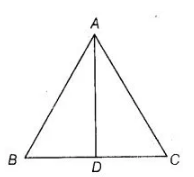# Find the altitude of an equilateral triangle of side 8 cm.

Question:

Find the altitude of an equilateral triangle of side 8 cm.

Solution:

Let ABC be an equilateral triangle of side 8 cm i.e., AB = BC = CA = 8 cm. Draw altitude AD which is perpendicular to BC. Then, D is the mid-point of BC.$\therefore \quad B D=C D=\frac{1}{2} B C=\frac{8}{2}=4 \mathrm{~cm}$

Now, $\quad A B^{2}=A D^{2}+B D^{2} \quad$ [by Pythagoras theorem]

$\Rightarrow \quad(8)^{2}=A D^{2}+(4)^{2}$

$\Rightarrow \quad 64=A D^{2}+16$

$\Rightarrow \quad A D^{2}=64-16=48$

$\Rightarrow \quad A D=\sqrt{48}=4 \sqrt{3} \mathrm{~cm}$

Hence, altitude of an equilateral triangle is $4 \sqrt{3} \mathrm{~cm}$.